# Algebra II : Graphing Parabolas

## Example Questions

### Example Question #3 : Graphing Quadratic Functions

All of the following are equations of down-facing parabolas EXCEPT:

Possible Answers: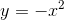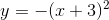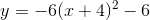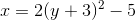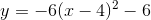Correct answer:Explanation:

A parabola that opens downward has the general formula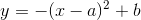,

as the negative sign in front of the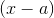term makes flips the parabola about the horizontal axis.

By contrast, a parabola of the form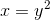rotates about the vertical axis, not the horizontal axis.

Therefore,is not the equation for a parabola that opens downward.

### Example Question #4 : Graphing Quadratic Functions

Consider the equation: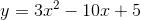The vertex of this parabolic function would be located at:

Possible Answers: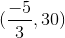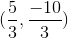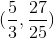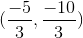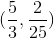Correct answer:Explanation:

For any parabola, the general equation is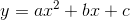, and the x-coordinate of its vertex is given by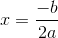.

For the given problem, the x-coordinate is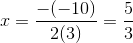.

To find the y-coordinate, plug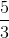into the original equation: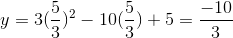Therefore the vertex is at.

### Example Question #5 : Graphing Quadratic Functions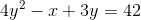In which direction does graph of the parabola described by the above equation open?

Possible Answers:

right

down

left

up

Correct answer:

right

Explanation:

Parabolas can either be in the form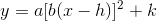for vertical parabolas or in the form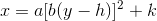for horizontal parabolas. Since the equation that the problem gives us has a y-squared term, but not an x-squared term, we know this is a horizontal parabola. The rules for a horizontal parabola are as follows:

• If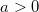, then the horizontal parabola opens to the right.
• If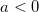, then the horizontal parabola opens to the left.

In this case, the coefficient in front of the y-squared term is going to be positive, once we isolate x. That makes this a horizontal parabola that opens to the right.

### Example Question #1 : Graphing Quadratic Functions

Find the vertex form of the following quadratic equation: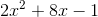Possible Answers: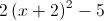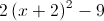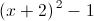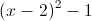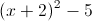Correct answer:Explanation:

Factor 2 as GCF from the first two terms giving us: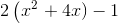Now we complete the square by adding 4 to the expression inside the parenthesis and subtracting 8 ( because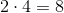) resulting in the following equation: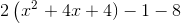which is equal to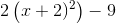Hence the vertex is located at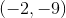### Example Question #7 : Graphing Quadratic Functions

Possible Answers:

Purple line

Blue line

None of them

Green line

Red line

Correct answer:

Red line

Explanation:

A parabola is one example of a quadratic function, regardless of whether it points upwards or downwards.

The red line represents a quadratic function and will have a formula similar to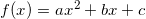.

The blue line represents a linear function and will have a formula similar to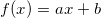.

The green line represents an exponential function and will have a formula similar to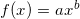.

The purple line represents an absolute value function and will have a formula similar to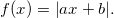.

### Example Question #8 : Graphing Quadratic Functions

Which of the following parabolas is downward facing?

Possible Answers: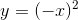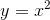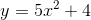Correct answer:Explanation:

We can determine if a parabola is upward or downward facing by looking at the coefficient of theterm. It will be downward facing if and only if this coefficient is negative. Be careful about the answer choice. Recall that this means that the entire value inside the parentheses will be squared. And, a negative times a negative yields a positive. Thus, this is equivalent to. Therefore, our answer has to be### Example Question #9 : Graphing Quadratic Functions

What is the vertex of the function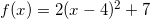? Is it a maximum or minimum?

Possible Answers: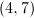; minimum; maximum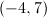; maximum; minimum

Correct answer:; minimum

Explanation:

The equation of a parabola can be written in vertex form: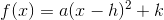.

The point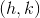in this format is the vertex. Ifis a postive number the vertex is a minimum, and ifis a negative number the vertex is a maximum.In this example,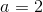. The positive value means the vertex is a minimum.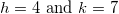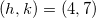### Example Question #1 : Graphing Parabolas

How many-intercepts does the graph of the function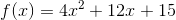have?

Possible Answers:

One

Two

None of these

Zero

Four

Correct answer:

Zero

Explanation:

The graph of a quadratic function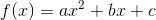has an-intercept at any point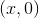at which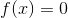, so, first, set the quadratic expression equal to 0: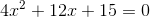The number of-intercepts of the graph is equal to the number of real zeroes of the above equation, which can be determined by evaluating the discriminant of the equation,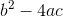. Set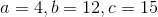, and evaluate: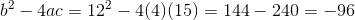The discriminant is negative, so the equation has two solutions, neither of which are real. Consequently, the graph of the functionhas no-intercepts.

### Example Question #131 : Functions And Graphs

Which of the following graphs matches the function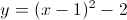?

Possible Answers: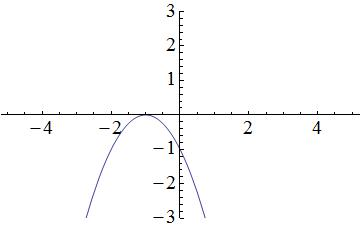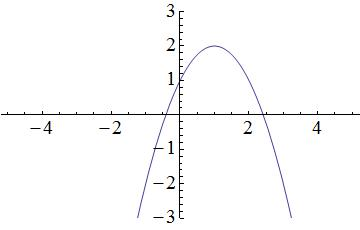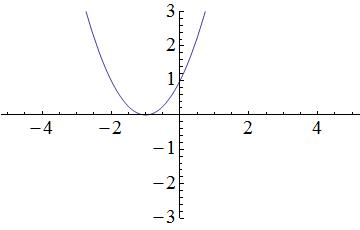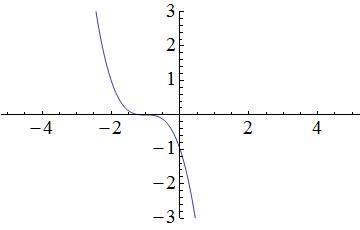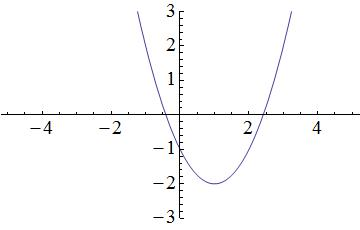Correct answer:Explanation:

Start by visualizing the graph associated with the function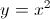: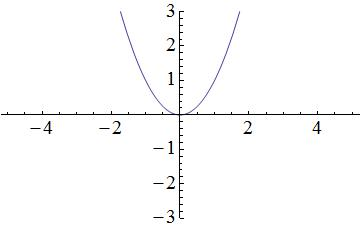Terms within the parentheses associated with the squared x-variable will shift the parabola horizontally, while terms outside of the parentheses will shift the parabola vertically. In the provided equation, 2 is located outside of the parentheses and is subtracted from the terms located within the parentheses; therefore, the parabola in the graph will shift down by 2 units. A simplified graph of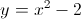looks like this: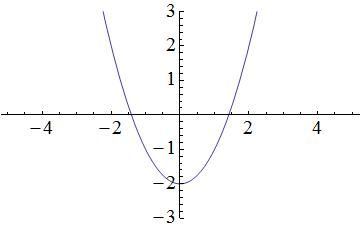Remember that there is also a term within the parentheses. Within the parentheses, 1 is subtracted from the x-variable; thus, the parabola in the graph will shift to the right by 1 unit. As a result, the following graph matches the given function:### All Algebra II Resources